# Measurement of Ship's Motions

### Mathematical Analysis

#### Consider an ELECTROLEVEL sensor mounted on board ship at a height h and lateral displacement b from the axis of a rolling motion represented by q = q1 sin w t (see figure 1). The polar coordinates of the sensor are R q, and the corresponding cartesian coordinates are X=R sinq    Y=R cos qq = q0+ q 1 sin w t An ELECTROLEVEL subject to accelerations x" ,y" produces an output which is interpreted as an effective angle qeff where qeff=qtrue - arctan(x"/(g+y"))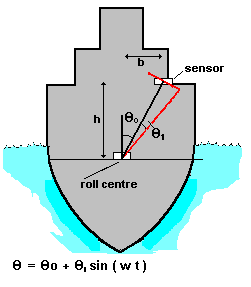Figure 1

#### Fig 2 shows such an arrangement where the roll centre of the ship is inaccessible. Two sensors are mounted at heights ha hb above the roll centre (where b = 0) The measured angles are thus: q a = q1 (1+ha w 2/g) sin (w t)qb = q1 (1+hb w 2/g) sin (w t)And the combination(hb qa - ha qb.)/( hb - ha )= q1 sin (w t) is the true roll angle. Note that error cancellation is frequency independent and hence applies for any oscillatory roll motion experienced by the ship. Practical limits to error cancellation techniques like this are determined by (a) flexure of the ship (b) the matching of the two sensor characteristics over their operating range.(This can be made quite close by selecting the sensors.) (c) third order effects caused by coupling of surge, yaw, heave and sway motions. The complexity of the latter are such that a particular application should be evaluated by computer simulation if high accuracy measurements are to be made.

Figure 2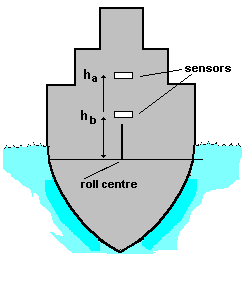### Dynamic response of the ELECTROLEVEL

#### To a first approximation the ELECTROLEVEL behaves like a simple pendulum with a natural frequency of oscillation fn and damping factor d. The natural frequency of the standard ELECTROLEVEL sensors is between 1 and 2.5 Hz and is ideally suited to following low frequency wave-induced motion. The damping factor d is between 0.6 and 0.9 at room temperature. Exceptionally, for a very small vessel a correction might have to be made for higher frequency oscillations .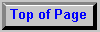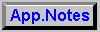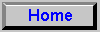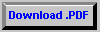Download Data Sheet as .PDF File (33Kbytes)